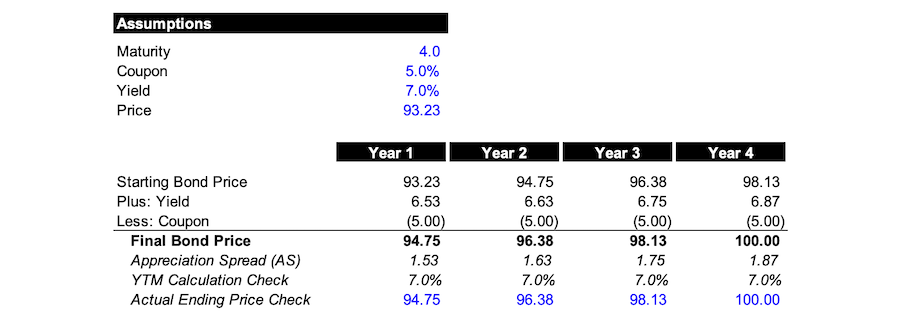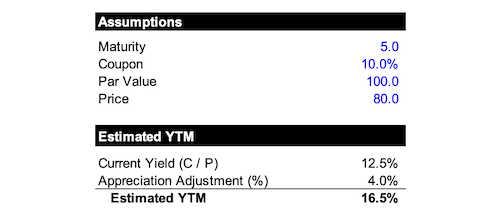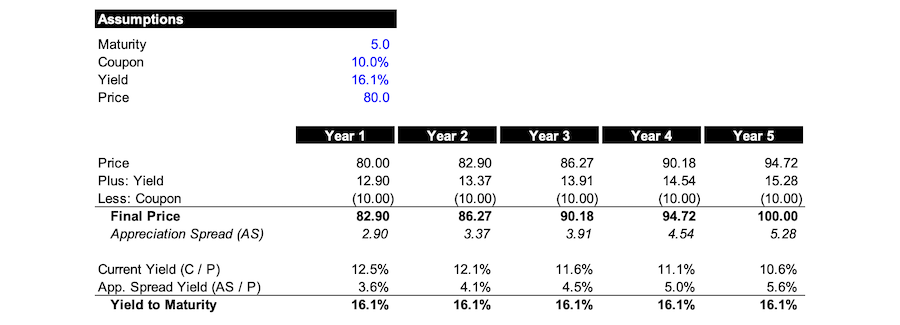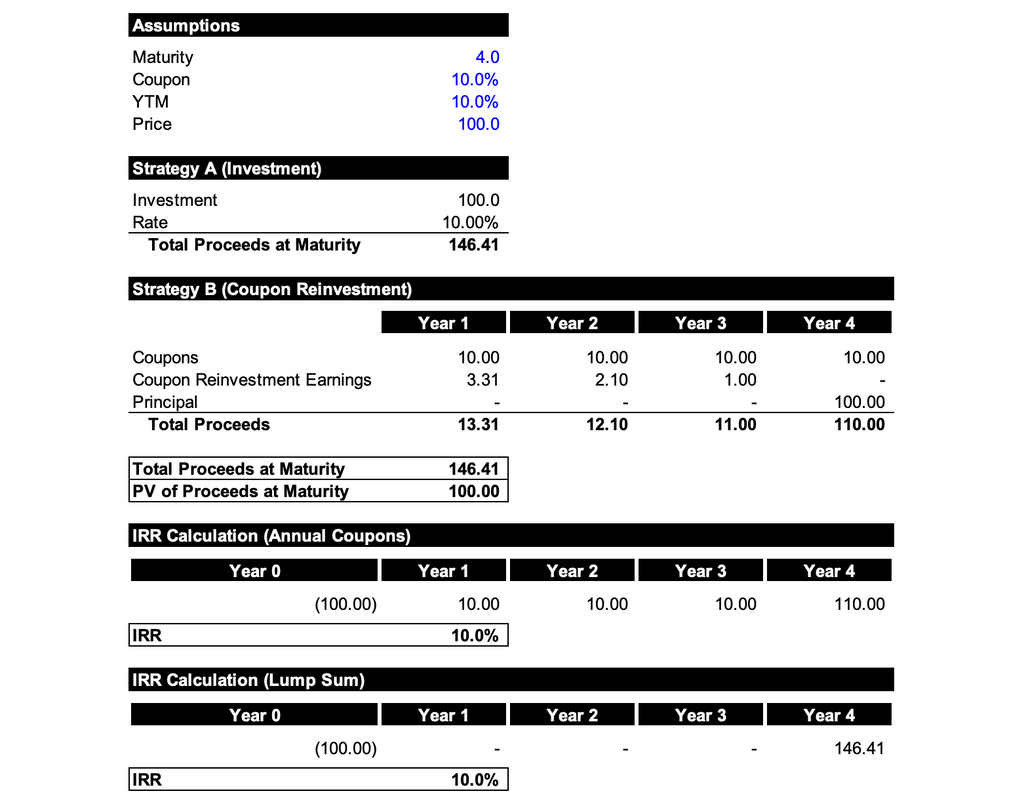# Bond Math Interview Questions: Some Things Worth Knowing

As you know if you’ve gone through the guides or have read enough posts around here, a relatively common type of restructuring interview question revolves around finding the YTM when given the coupon rate, years to maturity, and current trading price.

So, since recruiting season is upon us, I figured that I’d take a break this month from doing slightly more complicated posts on uptiers, cramdowns, death traps, etc. to discuss yields more generally and try to help build up your intuition a little.

Because even though there typically won’t be a wide variety of bond math questions in an interview, it’s never a bad idea to have a bit more of an intuitive understanding in case your interviewer wants you to eschew doing any math (e.g., using the estimated YTM formula) and explain things more extemporaneously.

To that end, I’ve put together a few questions below that will hopefully help prompt your thinking in the right direction and have tried to explain things in a more generalized way even if that’s at the cost of glazing over some nuance.

However, if you’re short on time and long on interview-related stress, the primary question that routinely comes up is the second question below, and you can answer it with either the estimated YTM formula or the more “intuitive” shortcut discussed in the answer here.

Just keep in mind that whenever you have a bond math question in an interview you’ll never actually be expected to do any convoluted calculations or need to explain any more cumbersome concepts (e.g., convexity). Instead, everything will usually be kept quite surface level and your interviewer is usually just looking for you to demonstrate a handwavy, directional understanding of what’s going on.

Anyway, to set the stage a bit, there are a number of different ways you can think about framing what a YTM is really saying. The most common (academic) framing is that it’s the rate at which you can discount the future cash flows (coupons and principal) of a bond so that the present value of those cash flows ends up aligning with the current price.

So, for example, if you have a bond with four years to maturity, a 5% coupon, and a yield of 7% then you know the current price should be 92.23. Below you can see that if you just discount the cash flows you’re getting (e.g., \$5 in coupons a year, \$100 at maturity) using the yield as your discount rate then you’ll end up with a PV of 93.23. Alternatively, if we pretend the coupon is equal to the yield (e.g., both 5%) we get a PV of 100 as we’d expect.

Note: If we’re holding the bond to maturity and reinvesting the coupons clipped at the YTM rate then our return (IRR) should equal the YTM, which is also what we find below.This is all well and good. But it perhaps doesn’t build that much of an intuitive understanding – beyond illustrating how as the YTM increases the price decreases – so let’s see if we can circle around a few more creative ways of thinking about all this.

## Let’s imagine we have a bond with four years to maturity, a 5% coupon, and a 7% yield that’s trading at 93.2 and will return par. How should the price of the bond change as it approaches maturity and how does the yield intuitively inform it?

This isn’t the kind of question that’d actually come up in an interview but since you’ll occasionally get more conceptual bond math questions – that get beyond just using the estimated YTM formula – let’s use this question as an excuse to take a step back for a second, eschew doing any real math, and think about bond pricing more intuitively.

Whenever you depart from relying on real math and begin trying to explain things more generally, you’ll inherently lose some nuance. But here’s one way you can think about yield in relation to bond pricing at a (maybe!) more intuitive level: it’s reflecting the amount the bond needs to return in a given year, based on its current price in a given year, to get it on a pathway towards being worth par at maturity.

This is something that many initially overlook, even though it’s obvious in retrospect: if you have a bond trading below par with a number of years until maturity and we say the yield is X%, then the value of that bond must increase as maturity approaches if the yield is to stay static through the period. Because if the bond weren’t to increase in value then the natural consequence would be for the yield to inch upwards as maturity approaches.

To put some meat on the bones, in our little example here we’ve been told a bond has four years to maturity, a coupon of 5%, and a yield of 7%. Consequently, the price of the bond will be around 93.2 assuming a par value of 100 (assume annual coupons).

If we were to plot the four years until maturity, what we should observe is that the price of the bond increases incrementally each year as we approach maturity until it reaches par while the actual yield stays static.

The obvious question then is how much should we theoretically expect the bond to increase each year. To answer that, let’s think about what yield is representing once again: it’s the return of the bond necessary to get it back to being worth par at maturity. Therefore, for a bond trading below par, that’ll necessitate there being a return in excess of the coupon rate because the price of the bond needs to “catch up” to the par value.

So, the theoretical amount the bond should increase in value in a given year should be reflective of the dollar (not percentage) spread between the return of the bond in a given year, based on the current price, and the coupon (which will obviously be a static value).

If this all seems a bit fuzzy, think about the little table below...We begin by taking our purchase price of 93.23 (based on there being a coupon of 5%, a yield of 7.0%, and four years to maturity). Applying the yield of 7.0% to the purchase price, that means the bond itself, in year one, “earns” a return of around 6.53. Stripping out the coupon component paid, this means the bond has 1.53 of price appreciation, and should theoretically be worth 94.751.

Now if you were to go use a yield to maturity calculator and type in a coupon of 5%, three (not four!) years to maturity, and a current trading price of 94.751 you’d find the yield to maturity is, as you’d guess, equal to 7.0%. Likewise, if you just play around with a YTM calculator and try to get a YTM of 7.0% by rejiggering prices around, you’ll hone in on, for example, 96.384 if you put a coupon of 5% and say there are just two years to maturity.

If you then tally up what I’m calling here the “appreciation spread” for all four years (e.g., the spread between the amount of return in a given year after stripping out the coupon component) you’ll get 6.77. And, since our initial purchase price was 93.23, adding these values together gets you 100 at maturity which must be the value of the bond at maturity.

Therefore, we’ve proven in our own circuitous way that the final value of the bond should be par (100) if it grows by 7% a year and, from an investor’s perspective, each year your effective return from owning the bond is per se going to be informed by having bought the bond below par.

So, glossing over some nuance, the takeaway here is that one way to think about yield when a bond is trading below par, from a slightly different perspective, is that it’s reflecting the return necessary for a bond to reach a par value at maturity and that it will, obviously, be an amount in excess of the coupon rate as it’s trying to “catch up” to par.

## Let’s say we have a bond maturing in five years with a 10% coupon that’s currently trading at 80 and will return par. Can you tell me, using as little math as possible, what the YTM roughly is?

Here we have all the ingredients needed to use the estimated YTM formula and, if we were to use it, we’d end up quickly getting 14 / 90 or 15.56%.

But let’s pretend that your interviewer balks at your utilization of the estimated YTM formula and says they want you to give a more “intuitive” answer. In this case, hopefully the rather lengthy question above helps prompt your thinking in the right direction.

Because what we found through the prior question is that a simplified way we can think about a bond trading below par is that its YTM is being informed by two components: the coupon component and the price appreciation component.

So, the way your interviewer will want you to answer this question is by thinking about these components separately…

First, we know that our YTM will be driven partly by the coupons we’re clipping (in this case, \$10 a year). But how favorable those coupons look is inherently contingent on the price that the bond is currently trading at (e.g., what you’re paying today to get this future stream of cash flows).

Therefore, a way to think about the portion of our YTM being driven by the coupons we’re clipping, and their subsequent reinvestment until maturity at the YTM rate, can be represented by the current yield (C / P) or, in this case, 10/80 = 12.5%.

Second, we know that our YTM will be driven partly by the price appreciation that’s occurring since we’re buying the bond below par and assuming it returns par in five years. As we saw in the prior example, the amount the bond will increase per year won’t be constant, but the amount of price appreciation each year, when tallied together, will equal the spread between the purchase price and par. In other words, the bond will reach par at maturity, it just won’t be going up each year by the exact same amount.

Therefore, a simplified way we can estimate the portion of our YTM that’s being driven by price appreciation is that it’s just the spread between par and the purchase price divided by the number of years until maturity.

In this case, it’ll be (100-80) / 5 = 4. So, we can say that we’re getting roughly 4% worth of principal pickup (price appreciation) per year.

Adding these two YTM components together we get 12.5% + 4% or 16.5%. Needless to say, this approach isn’t really much quicker than using the estimated YTM formula – but it does allow you to show a more intuitive understanding of what’s driving the YTM.The real YTM here is 16.126% (assuming annual coupons) and, if you’re curious, you can get a feel for how this bond changes as maturity approaches by revamping our quick-and-dirty analysis from the prior question...## Let’s say that we have two bonds. The first matures in five years with a 10% coupon and is trading at 80. The second matures in four years with a 10% coupon and is also trading at 80. What one do you think has a higher YTM?

If we use our strategy from the question above – eschewing the “real math” of the estimated YTM formula in favor of a more “intuitive” approach – then we’ll find that the first bond has a YTM of 16.5% while the second bond has a YTM of 17.5%.

In both cases the current yield (C/P) is identical. But in the case of the former we’re getting \$20 of price appreciation in five years, whereas in the latter we’re getting it in just four years.

So, it makes perfect sense that the shorter-duration bond should have a higher YTM because, all else being equal, it’s obviously better to get the same amount of cash sooner rather than later.## Let’s say that I didn’t believe that YTM actually assumes coupons are being reinvested at the YTM rate. How could you try to convince me it’s actually true?

This wouldn’t be asked in an interview context as it’s a little too conceptual. But working through this question will (hopefully!) allow us to put a bow on all of this yield talk and give you a reasonably intuitive understanding of the basics.

Note: In the future maybe I’ll put together a few questions on thinking through duration and convexity. But we’ll save that for a later day since no one is going to ask you the intuition behind convexity declining as yields increase in an interview.

Anyway, one way to show a coupon-reinvesting-skeptic the error of their ways would be to lay out the cash flows: take your coupons each year, compound them at the YTM rate for the relevant number of years left until maturity, tack on the principal you’re getting at maturity, and sum up the proceeds.

Then you could compare the total proceeds found to if you had just placed an amount of money, equivalent to the current trading price of the bond, into a bank account that promised to pay you an annual rate equivalent to the YTM for the number of years until maturity.

In both instances you’ll find that the total proceeds are equivalent and that their present value (when using the YTM as the discount rate) will bring you back to the current price.

Further, your return (IRR) will be the same whether you’re assuming you get the coupons each year and par at maturity or if you’re assuming you get no coupons and a lump sum of all the proceeds at maturity (keep in mind with the lump sum strategy that you’re assuming that you’re taking the coupons each year and immediately locking them into an investment paying the YTM rate for however many years until maturity – consequently, all proceeds arrive effectively only at maturity).

In the end, the YTM must assume that the coupons are being reinvested at the YTM rate. Because if the coupons weren’t reinvested at all, or were done so at some lower rate, then the PV of our total proceeds in Strategy B would be lower than the current price and the proceeds from Strategy A would be higher than the proceeds from Strategy B.

Note: Both of the IRR figures found below are equivalent to the YTM that we used (as they should be since the YTM should equal the IRR if you’re holding the bond to maturity and reinvesting coupons at the YTM rate).Just as a point of reference, if we assume that we have a bond trading at par – meaning that the coupon rate and YTM are the same – then, as you’d expect, we get an IRR that’s 10% regardless of if we do the lump sum method, or assume coupons are paid annually.## Conclusion

Hopefully this has helped flesh things out a bit for you. It can be easy to fall down the conceptual rabbit hole with bond math questions, but it’s important to remember that what folks refer to as “bond math” for interview purposes is a bit of a misnomer.

Rest assured, no one is going to be testing your mathematical limits in an interview. Instead, you’ll either get quite straight-forward quasi-math questions (e.g., finding the YTM as we did above) or you'll just be asked more conceptual questions where your interviewer will want you to give a more intuitive answer that eschews doing any real math.

So, for example, if you were asked if your return would be higher, lower, or the same if coupons were reinvested at a lower rate than the YTM then you now have a little mental model for explaining your answer. Because just think about the table from the prior question: the total proceeds would be lower if the coupons were reinvested at a lower rate than the YTM, so you’d expect the PV of the total proceeds to be lower than if coupons had been reinvested at the YTM.

Anyway, given that bond math questions will make up a relatively small part of an interview – as most interviewers want to focus on more restructuring-specific questions – it’s good to know the building blocks but you shouldn't spend an irrational amount on it.

Note: If you have upcoming superdays make sure to spend a little bit of time (but, just like with bond math, not an irrational amount of time!) developing answers to more general questions around if you think there will be a meaningful upswing in restructuring activity, what the impetus for increased activity will be, etc. In the members area there are a few research reports you can draw excellent talking points from, and there’s also the post I did several months ago on what will inform the next restructuring cycle that is still 100% applicable.